## Let's enjoy the computer vision using SAS Viya!

Started ‎03-28-2021 by
Modified ‎05-24-2021 by
Views 1,502
Paper 1016-2021
Authors

## Abstract

Image recognition technology enables machines to understand the content reflected in an image. Many studies in recent years have applied machine learning methods to computer vision problems. Among these methods, convolutional neural networks (CNN) is the most commonly used. CNN also has applications in other fields, such as natural language processing. Using CNN, higher-order features can be extracted by stacking layers that perform two different modes of processing. This report provides an illustration of the CNN structure. Further, it will address regions with CNN (R-CNN), used for object detection, and its successors, Fast R-CNN and Faster R-CNN, as examples of the application of CNN, as well as provide a schematic explanation of their processing flows. Lastly, this paper will introduce the program code of Faster R-CNN using SAS Viya(r). This paper will be helpful to those wishing to learn more about CNN as well as those who would like to attempt object detection using SAS Viya(r) for the first time, and will contribute to the development of future business and research activities.

## Watch the presentation

Watch our video presentation, Let's enjoy the computer vision using SAS Viya, on the SAS Users YouTube channel.

## Introduction

The difficulty in visualizing is that the information given is not enough to obtain a solution. Therefore, some model must be used to reach an optimal solution. Computer vision emerged in the early 1970s. It was considered to be responsible for vision in the process of making a computer imitate human intelligence and a robot behave intelligently. In other words, "vision" was thought to be just one simple step in solving difficult problems (Szeliski, 2010). Computer vision has evolved over time, and is now established as a field that automatically extracts visual information from given data, using techniques such as 3D modeling, image content search, and recognition and detection of target objects.

In recent years, machine learning methods have been applied to computer vision problems. Image recognition is one of the research fields dealt with in computer vision. It has been difficult for humans to try to make a computer process objects in the same way as they do. This is because in many cases, multiple objects are intricately intertwined in one image, and some objects, and even the same object looks much different depending on the angle and brightness.

Object recognition and object detection are related to image recognition and are similar in that they identify objects in images, but their purposes are different. Object recognition is the process of predicting the class of an object or the instance of an object in an image. "Predicting the class of an object" means predicting the concept to which the object belongs, such as dog, bridge, and building. "Predicting the instance of an object" is to predict the object itself, such as golden retriever, Golden Gate Bridge, and Empire State Building. On the other hand, object detection is a process that not only predicts the class or instance of an object in an image, but also estimates its position in the image. As a result of object detection, multiple objects appearing in one image are identified, and the position of each identified object is displayed in a square area.

The convolutional neutral network (LeCun et al.,1989; LeCun et al.,1998) is the most common of the methods that apply deep learning to image recognition. The method typically used before the emergence of CNN was bag-of-visual-words (Csurka, 2004). Image recognition using this method, also called bag-of-keypoints, begins with the detection of characteristic points in the image. These characteristic points are called keypoints or features. A small image is cut out around the keypoints, and patterns or distinct structures are extracted from the cut out image. The cut out image is called an image patch or patch, and the pattern or distinct structure is termed a local feature detector-descriptor. Local binary pattern (LBP) (Ojala et al., 1994), scale-invariant feature transform (SIFT) (Lowe, 2004), speeded-up robust features (SURF) (Bay et al., 2008), histogram of oriented gradients (HOG) (Dalal and Triggs, 2005), oriented FAST and rotated BRIEF (ORB) (Rublee et al., 2011), binary robust invariant scalable keypoints (BRISK) (Leutenegger et al., 2011), and AKAZE (Alcantarilla et al., 2013) are well known feature detectors and descriptors. The convolutional neutral network (CNN) is different from BoVW, and has been widely used in image recognition because of its ability to improve the rate of false positives.

CNN is a derivative of a deep neural network. A neural network is a computer imitation of the network of nerve cells in living creatures. A deep neural network is a deep network with many layers. In the medical field, CNN is being increasingly applied for image recognition. For example, Ardila et al. (2019) constructed a 3D-CNN model to detect cancer candidate regions using lung computed tomography (CT) image data of 14,851 patients to train the network model. The authors reported that the area under the receiver operating characteristic curve of the constructed model was 0.944 for 6,716 national lung cancer screening trials, which was the highest level of performance at that time. In this paper, we explain CNN, summarize regions with CNN features (R-CNN), Fast R-CNN, and Faster R-CNN, and introduce the application of Faster R-CNN using SAS Viya®.

## CONVOLUTIONAL NEURAL NETWORK

### CONVOLUTION LAYER

We assume preparing three filters with a height and width of 3 (3 × 3) for an image consisting of 6 × 6 pixels and convolving them. Figure 1 below shows the convolution by the first filter (Filter 1), where x1,1 is the input data of the pixel (1, 1), x1,2 is the input data of the pixel (1, 2),…, and x6,6 is the input data of the pixel (6, 6). In Figure 1, we refer to the parameter w_(p,q,k)^((l)) as the weight of the position (p, q) in the k-th filter of the l-th layer, b_k^((l)) as the bias of k-th filter of the l-th layer, and a_(m,n,k)^((l)) as the weighted input of position (p, q) through the k-th filter of the l-th layer. From the target image, the nine pixels on the upper left are scanned using Filter 1 to obtain the weighted input of the first unit. Subsequently, the nine pixels shifted by one pixel are scanned using the Filter 1 to obtain the weighted input of the second unit. This shifting process is called stride, and the shifting width is the stride size. Striding a 6 × 6 image with a stride of one requires weighted inputs in a total of 16 units before scanning the nine pixels in the lower right. The units so obtained in this way are arranged on a plane in order to utilize spatial information. This unit group is called a feature map. The weighted input of the unit at the position (m, n) of the feature map k in the first layer obtained by convolution from the input layer is given as follows: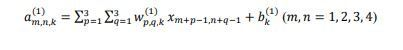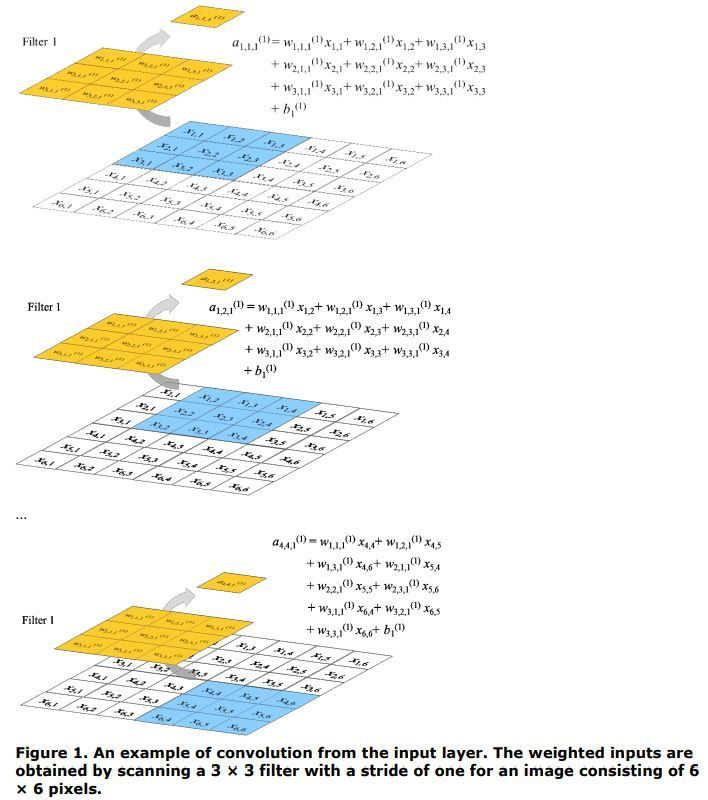By convolving the input layer using the second filter (Filter 2) and the third filter (Filter 3), we get the three feature maps as shown in Figure 2 below.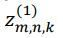is the output of the unit at the position (m, n) of the feature map in the first layer obtained by convolution from the input layer, obtained by convolution from the input layer, and is given as follows: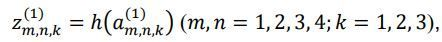where h is an activation function.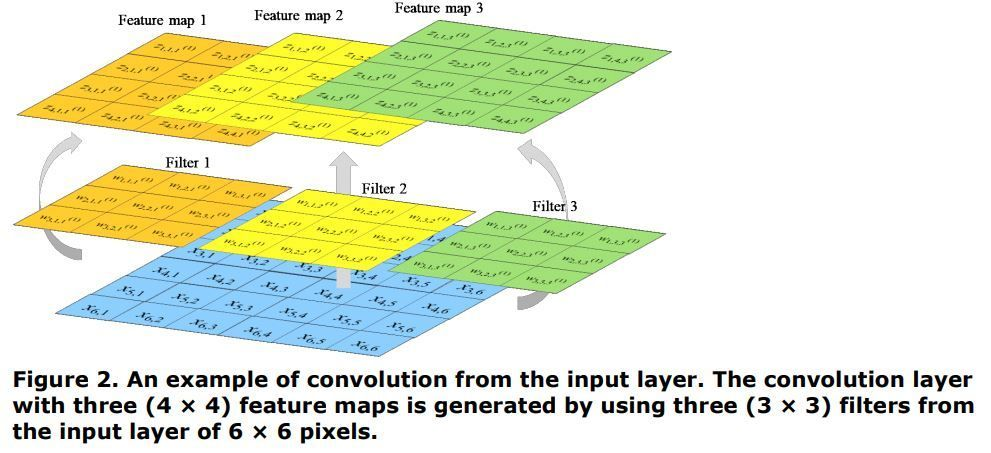Figure 3 below illustrates how the convolution layer shown in Figure 2 is convoluted using two filters (Filter 1 and 2). Since the first convolution layer has three feature maps, three filters are needed each for Filter 1 and Filter 2, when creating the second convolution layer from the first convolution layer. For each of feature map in the first convolution layer, nine pixels from the upper left are scanned by using Filter 1, and the weighted input of the first unit, , is obtained. Assuming a stride of one, a total of nine weighted inputs are obtained by scanning the pixels from the upper left to the lower right. Similarly for Filter 2, 9 pixels from the upper left for each of the feature maps are scanned, and the weighted input of the first unit is obtained. Four pixels from the lower right for each of feature map 1, 2 and 3 in the first convolution layer are scanned and the weighted input of the ninth unit are obtained. In Figure 3, z_(i,j,k)^((1)) is the output of the position (i, j) in the k-th filter of the first convolution layer, a_(m,n)^((s)) is the parameter of the position (m, n) in the s-th filter of the second convolution layer, and  w_(p,q,k,s)^((2)) is the weighted input of the position (p, q) through the k-th filter of the second layer is given, where b_s^(2) is the bias of s-th filter of the second convolution layer is given as follows: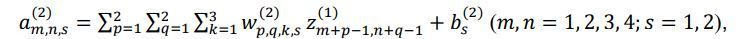where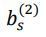is the bias of the s-th filter of the second convolution layer.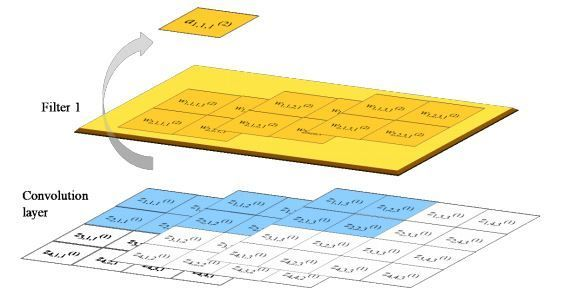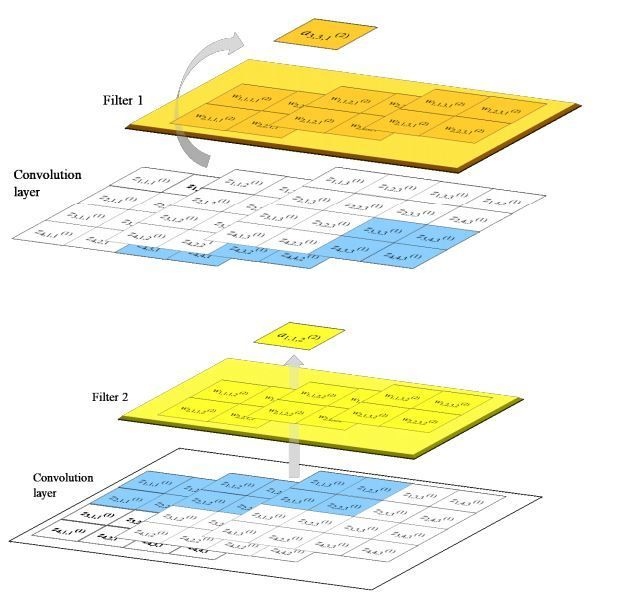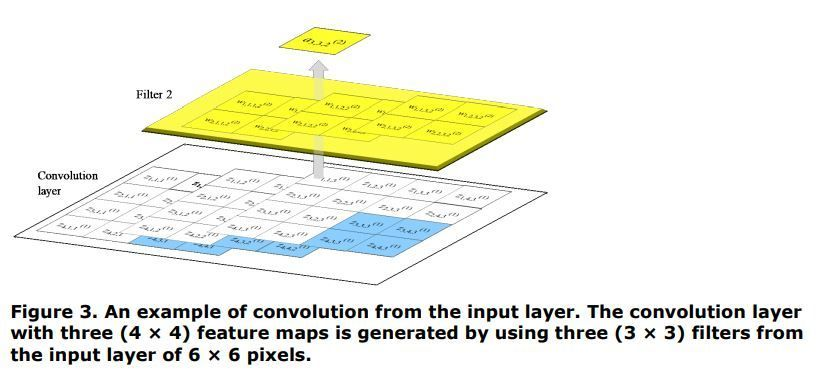Figure 4 below summarizes the convolution from the convolution layer shown in Figure 3. The second convolution layer has two feature maps of 3 × 3 obtained by convoluting Filter 1 and Filter 2, each with three filters of 2 × 2. The output of the position (m, n) in the s-th filter of the second convolution layer,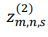, is given by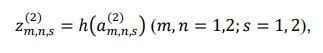where h is an activation function.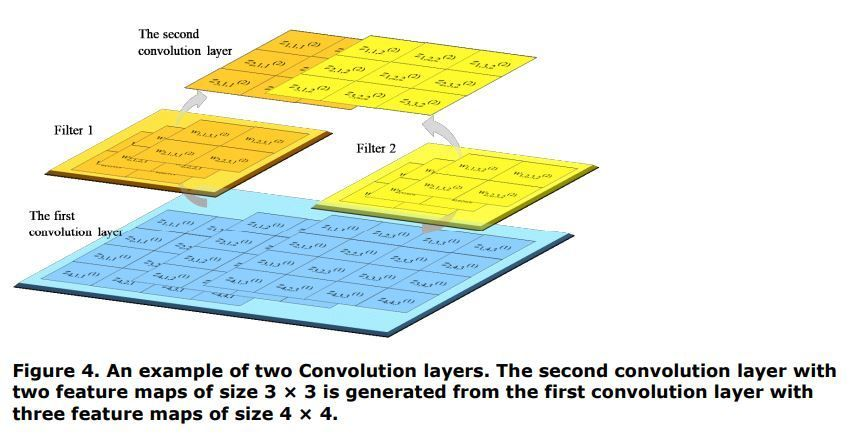### POOLING LAYER

Figure 5 below shows the results of the pooling by using the 2 × 2 pooling area with a stride of one for the convolution layer consisting of three feature maps of size 4 × 4 (feature maps 1, 2, and 3). Since a small area of size 2 × 2 is combined into one unit for each feature map of size 4 × 4, one layer with four units is formed from each feature map.  z_(i,j,k)^((l)) represents the input of unit at the position (i, j) of the feature map k in the l-th layer, and  z_(m,n,k)^((l+1)) represents the output of unit at the position (m, n) of the feature map  in the ( l+1)-th layer.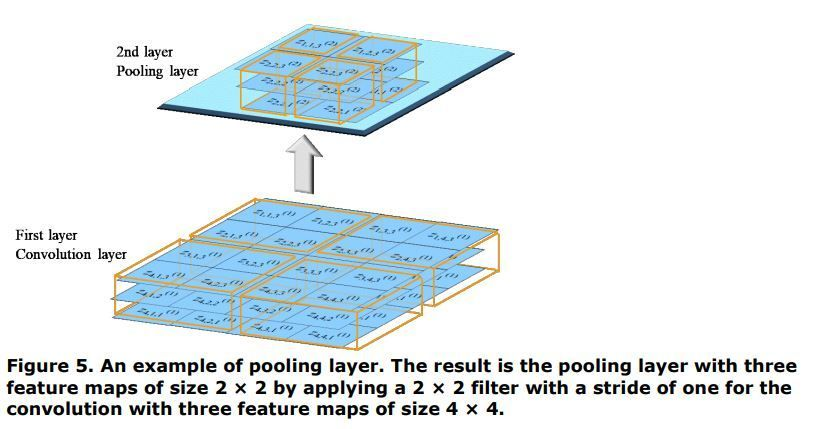The pooling layer has no weight parameter and no bias, and the output of the unit is equal to the input of the unit in the same pooling layer. Max pooling is the pooling that uses the maximum input value of the unit included in the small area for the pooling as the output value from the pooling layer, as follows: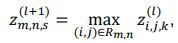where (m,n) is the location of the small area for pooling and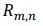is a set of units included in the small area for pooling.

Mean pooling is the pooling that uses the mean input value of the unit included in the small area for pooling as the output value from the pooling layer, as follows: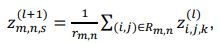whereis a cardinal number of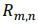.

Another method combines max pooling with mean pooling as the output value from the pooling layer.

## OBJECT DETECTION USING CNN

In object recognition, one object is shown in one image, and the aim is to recognize the object. On the other hand, in object detection, "what is where" is detected with a number of objects shown in one image. Object detection methods are basically of two types.

The first method involves predicting the area where the object is likely to be, and then identifying the class and position of the object. This method uses CNN, introduced in the previous section. R-CNN (Girshick et al., 2014) is the original. Fast R-CNN (Girshick, 2015) and Faster R-CNN (Ren et al., 2017) are proposed as advanced forms. The other method involves dividing the image into a fixed size and predicting the classification and position of the object class having a predetermined shape and size for each obtained area. You only look once (YOLO) (Redmon et al., 2016) and single-shot detector (SSD) (Liu et al., 2016) are some common techniques. The square border that surrounds the target object, such as an image or text, is called a bounding box. In YOLO, the image is first divided into several regions. For each divided region, the probability of the object class is calculated under the condition that the object exists in the region. Furthermore, the object confidence of the number of bounding boxes specified in advance is calculated for each region. The object confidence is calculated as the product of the probability that an object exists in the bounding box and an index providing how well the predicted bounding box matches the correct answer. Based on the probability of the object class under the condition that the object exists and the object confidence of each bounding box, processing is performed so that multiple bounding boxes are not selected for the same object, and the final object detection result is the output.

The former method is sometimes called the two-stage method because it first predicts the area where the object is likely to exist and then identifies the class and position of the object. The latter method is called the one-stage method. In this paper, we discuss the twostage method, illustrate R-CNN, Fast R-CNN, and Faster R-CNN, and introduce an application example of Faster R-CNN.

### REGIONS WITH CNN FEATURES (R-CNN)

The architecture of R-CNN (Girshick et al., 2014) consists of the following three stages.

• First stage

From the input image, multiple candidates for the area where the object seems to exist are extracted. Each such candidate area is called a region proposal. The selective search for object recognition is one method for extracting region proposals, and is performed as follows: divide the input image into several smaller areas and calculate the “similarity” between adjacent areas; select the pair of regions which are the most similar and combine them into a single large region; and repeat the process until all region proposals are extracted.

• Second stage

All the region proposals are resized to a fixed size and are provided as input to the already learned CNN. With the help of feature maps in the middle layer of the CNN, a class is assigned to the objects in the extracted region proposals using a classification algorithm (classifier), such as a support vector machine (SVM), and is scored.

• Third stage

When a region proposal with a score above a certain level is to be detected as a bounding box containing the target object class, there is a possibility of detecting multiple bounding boxes. This is because the extracted region proposals have regions that are slightly offset, and it is possible that the same object is included in those region candidates. Therefore, in order to prevent multiple bounding boxes from being detected for the same object, a score representing the reliability of detection is calculated for each bounding box, and only one bounding box is selected for the target object class by excluding the region where this score is the non-maximum value. This process is called non-maximal suppression (NMS). Finally, the shape of the bounding box is predicted, which is considered to depend on the class of the object. The coordinates, and height and width of the bounding box are set as the parameters of the bounding box, and regression using the feature map for these parameters is performed. (See Figure 6 below.)### FAST REGION-BASED CONVOLUTIONAL NETWORK METHOD (FAST R-CNN)

R-CNN (Girshick et al., 2014) is an excellent network method for object detection. However, as learning is performed in multiple stages, it takes time to execute and object detection is slow. Girshick (2015) proposed Fast R-CNN, a network that speeds up execution and enhances object detection performance.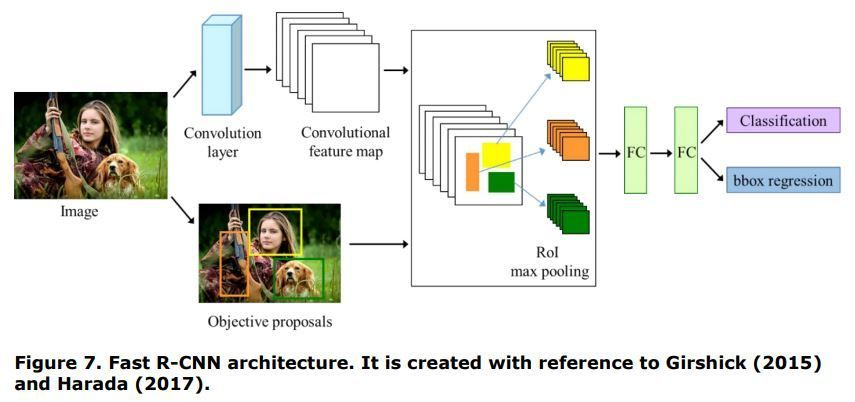Figure 7 above shows the architecture of Fast R-CNN. The image is fed as input to CNN and convolutional feature maps are obtained from the convolutional layer in the middle of the CNN. Objective proposals are extracted in parallel using methods such as selective search for object recognition. In the region of interest (RoI) layer, the feature map corresponding to the extracted objective proposal is cut out and converted to a fixed size, following which max pooling is performed. After passing the data through the fully connected layers, the posterior probability of the class is calculated, and regression to the bounding box is performed to obtain as output the coordinates, and width and height of the bounding box depending on the class.

One of the improvements in Fast R-CNN is the introduction of a RoI pooling layer. In the RoI pooling layer, max is performed on the feature map corresponding to the region proposal, and a fixed-size feature map is obtained. In Fast R- pooling CNN as well as R-CNN, multiple region proposals are extracted using selective search for object recognition method. In R-CNN, the feature map is obtained using the trained CNN for each region proposal. On the other hand, in Fast R-CNN, the image is fed as input to the trained CNN, and the convolutional feature map is obtained from the convolution layer in the middle of the CNN. The small feature map cut out from the convolutional feature map based on the information given in the region proposal is called the region of interest (RoI). In the RoI pooling layer, the RoI is divided into fixed-size areas (area with height  and width ), and maximum pooling works on the divided areas. For example, suppose the output size of the RoI is H =7, W =7, and the size of the feature map corresponding to the cut out region proposal is height h =21 and width w =14. The feature map corresponding to the region proposal cut out is divided into 3 × 2 windows ( h/H = 3,  w/W = 2), and the max pooling works on the obtained feature map with a size of 7 (= 21 / 3) × 7 (= 14 / 2). The resultant data is sent to the fully connected layer. The fully connected layer has two output layers, one that classifies using the softmax function and one that outputs the regression to the bounding box. The posterior probability of the class and the predicted coordinates, and width and height of the bounding box are output for the region proposal. The score indicating the object confidence is assigned using the class posterior probability output for each region proposal, and NMS is performed for each class of the object.

Another improvement in Fast R-CNN is that it performs classification and regression to the bounding box in parallel with the learning. When K different classes are detected, for each RoI from the output layer of Fast R-CNN, the posterior probabilities of the class, p = (p1,…,pK), and the parameters of the bounding box labeled with class u, t_^(u)= (t_x^(u) ,t_y^(u) ,t_h^(u) ,t_w^(u) ) , are the output. For the training input of learning, given the parameters of the bounding box labeled with ground-true class u, the multi-task loss L is defined. λ is the hyper parameter for the balancing the classification loss and regression loss. Girshick (2015) compared the performance of Fast R-CNN and R-CNN, where the mean and variance of  were set to 0, and  = 1 for Fast R-CNN.

### FASTER R-CNN

Faster R-CNN (Ren et al., 2017) introduced a region proposal network (RPN), and reduced the cost of the object detection to implement a module that extracts the region proposal and a module that performs class prediction of the object and regression to the bounding box of the object in a single network.

The image is fed as input to the CNN, and convolutional feature maps are obtained as output from the last convolution layer of the CNN. A small window with size n × n (this is written as "sliding window" in the original paper) is slid on the convolutional feature maps, and small regions are generated. In practice, small regions are generated by convolution using a filter of size n × n and a filter of size 1 × 1 for the convolutional feature maps obtained from the last convolution layer of the CNN. In RPN, we predict the presence or absence of an object for each small region obtained by using the sliding window on the convolutional feature maps. To present the output information for the object, "anchor" boxes are introduced. The anchor boxes are generated by using the base size, scale, and aspect ratio of the anchor.

Figure 8 below shows the anchor boxes on pixel (4, 5) pixel (fourth from the top and fifth from the left) in the convolutional feature map. The anchor scales are set as 8, 16, and 32, and 1:1, 1:2, and 2:1 are set as the aspect ratios of the anchor; ■ in the figure is a base anchor box. Anchor boxes of scale 8, 16, and 32 with the three aspect ratios (1:1, 1:2, and 2:1) are displayed in red, blue, and green, respectively. Since three patterns of the aspect ratios are set, there are three red, three blue, and three green anchor boxes.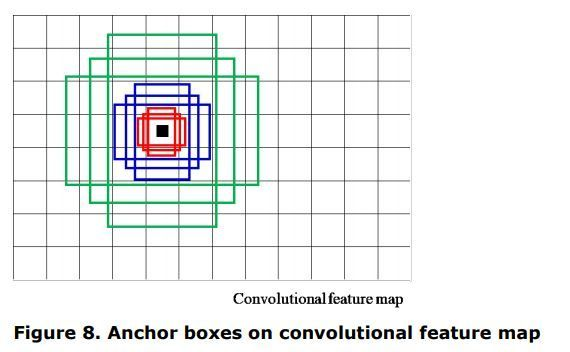In the RPN, it is predicted whether each of the W×H×k1×k2 anchors generated is an object. An intersection over union (IoU) is used to predict whether or not is an object. If the estimated IoU from the given data is less than 0.3, the anchor is labeled as the background, and if the IoU is greater than 0.7, it is labeled as an object (Ren et al., 2017). For example, assuming nine patterns of anchor, the result of predicting whether or not the anchor is an object is provided as a vector having 2 × 9 = 18 components for each sliding position on the convolutional feature map. For an each anchor box to be detected in an object class, a total of four parameters are predicted, including coordinates (x, y) and size (width and height). For example, assuming nine patterns of anchor, the prediction result regarding the object class information is given as a vector having 4×9 = 36 components for each sliding position on the convolutional feature map. All vector components of the object detected background class are given as zero vector.

Figure 9 below shows the architecture where an image is fed as input to the CNN and the prediction of whether or not it is an object class and the prediction regarding the object information are obtained as output for each anchor box. In the region proposal layer, a box-classification layer (cls layer) and a box-regression layer (reg layer) are obtained as the output. The cls layer gives the scores that estimate the probability of whether the anchor is an object or not. The reg layer outputs the coordinates and size of the object. The image is provided as input to the CNN, and convolutional feature maps are obtained as output from the convolution layer of the CNN. The dimensions of the convolutional feature maps are reduced using a sliding window. The anchor boxes are generated on the convolutional feature maps. For each anchor box, it is predicted whether the content is an object. The coordinates and size of the object are predicted simultaneously.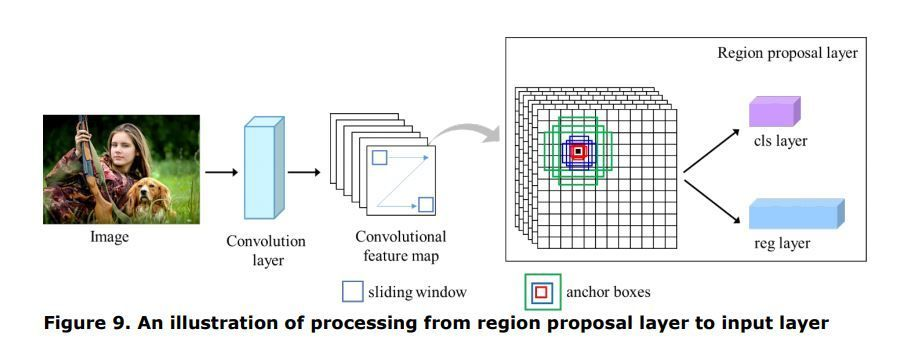In the RoI pooling layer, following the region proposal layer, the feature maps for the RoI are extracted using the estimated probability of whether the anchor box is an object or not, and the coordinates, and width and height of the object for each anchor box. By dividing the extracted feature maps into several areas and adding max pooling, we obtain multiple feature maps of a certain size. These feature maps are aggregated in one dimension through the fully connected layer. The fully connected layer branches into two output layers: one layer outputs the predicted probabilities over the object classes and a background class, and the other layer outputs four parameters of the predicted bounding box. For example, in the case of 20 object categories, the number of output units in the classification layer is 20＋1 = 21, and that in the bounding box layer is 4 × (20＋1) = 84. This is because we predict a background class in addition to the object classes.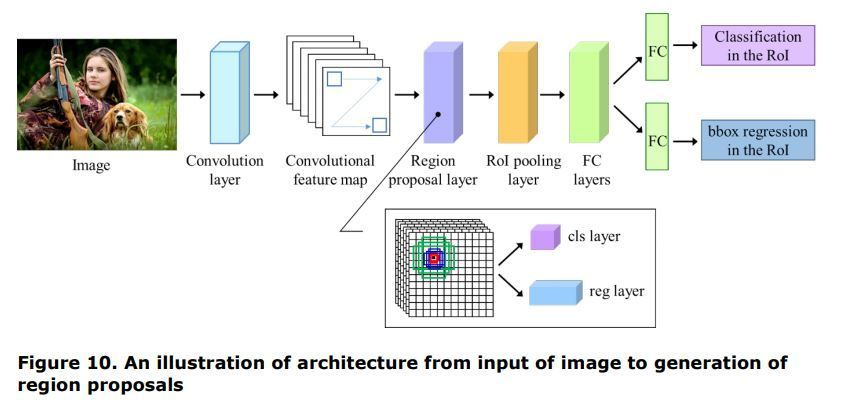Figure 10 above illustrates the architecture from the point the image is fed as input to the CNN to generation of revision proposals. The network has the ability to output the feature map from the learned CNN; output a set of object proposals, each with an object class score; and predict the object class and the location of the object. The image is fed as input to the CNN and the convolutional feature maps are obtained as output from the convolution layer of the CNN. In the region proposal layer, the presence or absence of an object is predicted for feature maps and information about the location of the object using anchor boxes. In the RoI pooling layer, the feature maps are extracted for the RoI. The feature maps passed through the fully connected layer are fed as input to two layers (classification in the RoI and bounding box regression in the RoI).

The multi-task loss is calculated for the two task layers, the region proposal layer and the Fast R-CNN layer. The multi-task loss in the Fast R-CNN layer is similar to the multi-loss in the region proposal layer. However, there are some differences in multi-task loss between the two layers.

• For multi-task loss in the region proposal layer, the anchor generated in the region proposal layer is used as an element (the subscript i in the definition formula of multi-task loss is the i-th anchor generated in the region proposal layer). On the other hand, the element of multi-task loss in the Fast R-CNN layer is the RoI output from the region proposal layer (the subscript i  in the definition formula of multi-task loss in Fast R-CNN is the i -th RoI output from the region proposal layer).
• For multi-task loss in the region proposal layer, the object is classified by the IoU of the anchor and the true bounding box. On the other hand, for multi-task loss in the Fast R-CNN layer, the object is classified by the IoU of the predicted object bounding box and the true bounding box.
• In the region proposal layer, the element of classification loss is L_cls (p_i,p_i^* )=-p_i^*lnp_i-(1-p_i^*)ln⁡(1-p_i) . On the other hand, the element of classification loss in the Fast R-CNN layer is L_cls (p_i,p_i^* )=-ln⁡(p_i^c) , where p_i^c is the predictive probability of the region proposal for the object class .

### STEP BY STEP IMPLEMENTATION OF FASTER R-CNN

Faster R-CNN is supported in SAS® Visual Data Mining and Machine Learning (VDMML) 8.4. VDMML is based on SAS Viya® which is a SAS platform. In the Deep Learning Programming Guide of VDMML, the SAS program code for classifying 20 types of objects by Faster R-CNN, which uses VGG16 as CNN and three patterns of anchor scales 8, 16 and 32, and anchor aspect ratios of 1:1, 1:2, 2:1, is introduced.

SAS® Cloud Analytic Services (CAS) provides ActionSet. Regarding deep learning, ActionSet includes functions that perform processing in each layer, such as the convolution layer, pooling layer and RoI pooling layer. By using these functions, a network model can be easily created and executed. Furthermore, SAS® Scripting Wrapper for Analysis Transfer (SWAT) which is a library that modularizes the function to call the Web API, and SAS® Deep Learning with Python (DLPy) package for Python API, which is prepared for deep learning and image recognition, are provided. By using SWAT and DLPy, it is possible to program CAS in a notation similar to Python. The source program code of Faster R-CNN using action set was introduced in the programming guide of SAS VDMML (SAS Institute Inc., 2019) and Xindian et al. (2019). The following program is an example of the code with detailed explanations that imports the required modules and builds Faster R-CNN. The example source code shows the Python notation. Programming using the R notation or CAS-specific languages (CASL) is also possible.

``````import swat /* Import SWAT package, which is a module that calls a Web API */
import dlpy.model import Model
from dlpy.layers import Detection
from dlpy.unitsimport *
from dlpy.images import ImageTable
nclasses = 2 ; /* Each anchor has 2 classes (an object class or a background class) */
anchorScaleV = [8,16,32] ; /* Set 3 scales of anchors to 8, 16, and 12 */
anchorRatioV = [0.5,1,2] ; /* Set 3 aspect ratios of anchors to 2:1, 1:1, 1:2 */
anchorNum = len(anchorScaleV)*len(anchorRatioV) /* Set yielding anchors */
classNum = 20 ; /*Set object classes (except a background class) to 20*/

/* Add a convolution layer with filters of size 3 × 3 and stride 1 × 1 */
model= mName, name = 'rpn_conv_3x3',
layer = dict(type = 'convolution', nFilters = 512, width = 3, height = 3,
stride = 1, act = 'relu'),
srcLayers = ['conv16'])``````

In the example source code, because of using the learned VGG16, the network processes a convolution layer with 512 filters, which channels the feature map output from the last convolution layer of VGG16 for the input layer named ‘conv16’. We use 512 filters of size 3 × 3, stride width (horizontal) 1, stride height (vertical) 1, and assign the activation function to a rectified linear unit (ReLU) and specify the name of the output layer as 'rpn_conv_3×3’.

``````/* Add a convolution layer with filters of size 1 × 1 and stride 1 × 1 */
model = mName, name = 'rpn_score',
layer = dict(type = 'convolution', nFilters = (nclasses+4)*anchorNum,
width = 1, height = 1, stride = 1, act = 'identity'),
srcLayers = ['rpn_conv_3x3'])``````

For the feature maps generated by convolution with filters of size 3 × 3 (the ‘rpn_conv_3x3’ layer), we create a convolution layer, named ‘rpn_score’, with filters of size 3 × 3 and stride 1 × 1. The reason behind defining ‘nFilters = (nclasses+4)*anchorNum’ in the example source code is that it predicts whether the image is an object is or not (that is ‘nclasses = 2’ in the example source code) and the four parameters including coordinates (x, y) and the width and height for each of the nine patterns of anchor boxes (three patterns as anchor scales and three patterns as aspect ratios).

``````/* Add a region proposal layer */
model = mName, name = 'rois',
layer = dict(type = 'REGIONPROPOSAL', act = 'identity',
coordType ='COCO', baseAnchorSize = 16,
anchorNumToSample = 256,
anchorScale = anchorScaleV, anchorRatio = anchorRatioV),
srcLayers = ['rpn_score'])``````

For the input layer named ‘rpn_score’, which is created by the convolution with the filters of size 3 × 3 and the convolution with the filters of size 1 × 1, we generate nine patterns of anchor boxes for the first pixel to the last pixel on the convolutional feature maps. For each anchor box, we output the predictive probability of whether it is an object, and the prediction result of its location information. In the example source code, we set the base anchor size to 16, and specify the name of the output layer as 'rois’. By using the anchorNumToSample = option, it is possible to specify the sampling number of anchor boxes for learning. The coordType = option selects the type for the detection results. If coordType = 'RECT', which is the default option, (xleft, ytop, width, height) is selected as pixel coordinates. If coordType = 'COCO’, (xmin, ymin, xmax, ymax) is selected as pixel coordinates.

``````/* Add an RoI pooling layer */
model = mName, name = 'pool5',
layer = dict(type = 'roipooling', poolWidth = 7, poolHeight = 7),
rcLayers = ['conv16', 'rois'])``````

Two input layers, where one is the layer that outputs from the last convolution layer of the learned CNN named ‘conv16’ and the other that outputs the region proposal layer named ‘rois’. By dividing the extracted feature maps for the RoI into several areas and adding max pooling, feature maps of a certain size are obtained. In the example source code, we set the size of the RoI to height  =7 and width  = 7. Thus, for example, in the case where the feature map for the RoI has height  = 21 and width  = 14, it is divided by a window sized 3 × 2 (  = 3,  = 2), and pooling works on the feature map sized of 7 (= 21 / 3) × 7(= 14 / 2).

``````/* Add 2 fully connected layers */
model = mName, name = 'fc6',
layer = dict(type = 'fullconnect', n = 4096, act = 'relu'),
srcLayers = ['pool5'])
model = mName, name = 'fc7',
layer = dict(type = 'fullconnect', n = 4096, act = 'relu'),
srcLayers = ['fc6'])``````

In the example source code, we added two fully connected layers. For both the layers, we assign the activation function to a ReLU, and set the number of units in the output layer to 4096.

``````/* Add a classification in the RoI */
model = mName, name = 'cls_score',
layer = dict(type = 'fullconnect', n = (classNum+1), act = ’identity’),
srcLayers = ['fc7'])``````

We add a layer that outputs confidence scores corresponding to the RoI. The number of units in the output layer is ‘n = (classNum+1)’. This is because there is a background class in addition to object classes (‘classNum' in Program 1) for the classification.

``````/* Add a bounding box regression in RoI */
model = mName, name = 'bbox_pred',
layer = dict(type = 'fullconnect', n = 4*(classNum+1), act = 'identity'),
srcLayers = ['fc7'])``````
We add a layer that outputs the regression to the bounding box in the RoI. The number of units in the output layer is ‘n = 4*(classNum+1)’, because we estimate four parameters (top corner, left corner, height, and width) for each set, and perform classification on the object in addition to the background.

``````/* Add a Fast R-CNN layer */
model = mName, name = 'fastrcnn',
layer = dict(type = 'fastrcnn', nmsIouThreshold = 0.3,
detectionThreshold = 0.7),
srcLayers = ['cls_score', 'bbox_pred', 'rois'])``````

Object detection works on an entire input image by using the bounding boxes and confidence scores (IoUs) in the region proposals. NMS prevents multiple areas from being selected for the same object. The nmsIouThreshold = option specifies the IoU threshold of the NMS. The detectionThreshold = option specifies the threshold for object detection.

## Conclusion

In this study, we discussed CNN as a deep neural network model and illustrated it graphically. As an application of CNN, we summarized R-CNN (an object detection method), and Fast R-CNN and Faster R-CNN (successors of R-CNN). The expressive ability of a network is said to be exponential with respect to the depth of the layer, whereas it is multinomial with respect to the width of the layer. In order to enhance the expressiveness of the network, it is more efficient to have multiple layers than to increase the number of parameters. It seems that these are the reasons for increasing the utility value of deep neural networks. The mathematical formulas used to describe the structure of networks certainly look esoteric, but most of them can be explained through linear algebra and infinitesimal calculus. We tried to visualize the structure of the network by using many figures so that the figures could be linked to mathematical formulas.

The most commonly used programming language in the field of machine learning is Python. SAS® is a minor programming language in the field of machine learning. However, fortunately, it has become possible to use programming languages other than SAS®, such as Python and R, with SAS Viya®. By using Python's artificial intelligence (AI) library via Jupyter Notebook supported by SAS Viya®, it is no longer necessary to program the processing at each layer of the network one at a time. SAS® Studio may be easier for SAS users because it allows them to program and execute machine learning techniques in the SAS language. For example, SAS Viya® provides the NNET procedure for running neural networks. By specifying the settings related to the network with regard to the build and the learning, it is possible to execute the neural network easily, and you can experience deep learning only in the SAS language. On the other hand, even in an environment where SAS Viya® cannot be used, SAS® can be linked with other analysis software such as Python and R.

Life forms on Earth underwent major evolution during the Cambrian period, and the development of eyes is said to be the triggering event behind this evolution (Parker, 2003). What it means for a computer to view an object, and whether computers can have abilities similar to or better abilities than humans, is of great interest to researchers studying the world of computer vision and implementing various techniques. SAS® can be helpful for these purposes. Perhaps computers will also undergo a period of rapid evolution as they acquire greater vision.

## References

Alcantarilla, P., Nuevo, J., and Bartoli, A. 2013. “Fast explicit diffusion for accelerated features in nonlinear scale spaces.” Proceedings of the British Machine Vision Conference, 13.1-13.11. BMVA Press.

Ardila, D., Kiraly, A., P., Bharadwaj, S., Choi, B., Reicher, J., J., Peng, L., Tse, D., Etemadi, M., Ye, W., Corrado, G., Naidich, D., P., and Shetty, S. 2019. “End-to-end lung cancer screening with three-dimensional deep learning on low-dose chest computed tomography.” Nature Medicine, 25: 954–961.

Csurka, G., Bray, C., Dance, C. and Fan, L. 2004. “Visual categorization with bags of keypoints.” Proceedings of European Conference on Computer Vision Workshop on Statistical Learning in Computer Vision, 59–74. Berlin, Heidelberg: Springer-Verlag.

Bay, H., Ess, A., Tuytelaar, T., and Gool, L., V. 2008. “Speeded-up robust features (SURF)”. Computer Vision and Image Understanding, 110: 346–359.

Triggs, B. and Dalal, N. 2005. “Histograms of oriented gradients for human detection.” Proceedings of the 2005 IEEE Computer Society Conference on Computer Vision and Pattern Recognition, 886-893. Washington, DC: IEEE Computer Society.

Fisher, R., A. 1936. “The use of multiple measurements in taxonomic problems.” Annals of Eugenics, 7: 179–188.

Girshick, R., Donahue, J., Darrell, T., and Malik, J. 2014. “Rich feature hierarchies for accurate object detection and semantic segmentation.” Proceedings of the 2014 IEEE Conference on Computer Vision and Pattern Recognition, 580-587. Washington, DC: IEEE Computer Society.

Girshick, R. 2015. “Fast R-CNN.” Proceedings of the 2015 IEEE Conference on Computer Vision and Pattern Recognition, 1440-1448. Washington, DC: IEEE Computer Society.

Glorot, X. and Bengio, Y. 2010. "Understanding the difficulty of training deep feedforward neural networks." Proceedings of the Thirteenth International Conference on Artificial Intelligence and Statistics, 249–256. Brookline, MA: Microtome Publishing.

Harada, T. 2017. Image Recognition (in Japanese). Tokyo: Kodansha.

Leutenegger, S., Chli, M., and Siegwart, R., Y. 2011. "BRISK: Binary robust invariant scale keypoints." Proceedings of the 2011 IEEE International Conference on Computer Vision, 2548-2555. Washington, DC: IEEE Computer Society.

LeCun, Y., Boser, B., Denker, J., S., Henderson, D., Howard, R., E., Hubbard, W., and Jackel, L., D. 1989. Backpropagation applied to handwritten zip code recognition. Neural Computation, 1: 541-551.

LeCun, Y., Bottou, L., Bengio, Y., and Haffner, P. 1998. “Gradient-based learning applied to document recognition” Proceedings of the 1998 IEEE International Conference on Pattern Recognition, 2278-2324. Washington, DC: IEEE Computer Society.

Liu, W., Anguelov, D., Erhan, D., Szegedy, C., Reed, S., Fu, C., Y. and Berg, A., C. 2016. "SSD: Single shot multiBox detector." Proceedings of the European Conference on Computer Vision, 21-37. Berlin, Heidelberg: Springer Science + Business Media.

Lowe, D., G. 2004. “Distinctive image features from scale-invariant keypoints.” International Journal of Computer Vision, 60: 91–110.

Minsky, M. and Papert, S., A. 1987. Perceptrons. Expanded Edition. Cambridge, MA: The MIT Press.

Nesterov, Y. 1983. “A method of solving a convex programming problem with convergence rate O(1/k2).” Soviet Mathematics Doklady, 27: 372–376.

Ojala, T., Pietikainen, M., and Harwood, D. 1994. “Performance evaluation of texture measures with classification based on kullback discrimination of distributions.” Proceedings of 12th International Conference on Pattern Recognition, 582-585. Washington, DC: IEEE Computer Society.

Parker, A. 2003. In the blink of an eye: how vision sparked the big bang of evolution. New York, NY: Basic Books.

Redmon, J., Divvala, S., Girshick, R. and Farhadi, A. 2016. “You only look once: Unified, real-time object detection.” Proceedings of the 2016 IEEE Conference on Computer Vision and Pattern Recognition, 779-788. Washington, DC: IEEE Computer Society.

Ren, S., K, He., Girshick, R., and Sun, J. 2017. “Faster R-CNN: Towards real-time object detection with region proposal networks.” IEEE Transactions of Pattern Analysis and Machine Intelligence, 39: 1137–1149.

Rublee, E., Rabaud, V., Konolige, K., and Bradski, G. 2011. “ORB: An efficient alternative to SHIFT or SURF.” Proceedings of the 2011 IEEE International Conference on Computer Vision, 2564-2571. Washington, DC: IEEE Computer Society.

Rumelhart, D., E., Hinton, G., E., and Williams, R., J. 1986. “Learning representations by back-propagating errors.” Nature, 323: 533–536.

Szeliski, R. 2010. Computer Vision: Algorithms and Applications. New York, NY: Springer.

Xindian, L., Maggie, D., and Xiangqian, H. 2019. “Exploring computer vision in deep learning: Object detection and semantic segmentation.” Proceedings of the SAS Global Forum 2019 Conference, Cary, NC: SAS Institute Inc.

## Acknowledgements

Shinjo Yada’s research was partially supported by the Support Program for Biostatisticians / Project Promoting Clinical Trials for Development of New Drugs and Medical Devices (20lk0201061t0005) from the Japan Agency for Medical Research and Development (AMED). Ryuji Uozumi's research was partially supported by a Grant-in-Aid for Scientific Research (20H04147) from the Ministry of Education, Culture, Sports, Science and Technology of Japan and the Practical Research Project for Allergic Diseases and Immunology (20ek0410069h0001) from AMED.